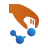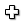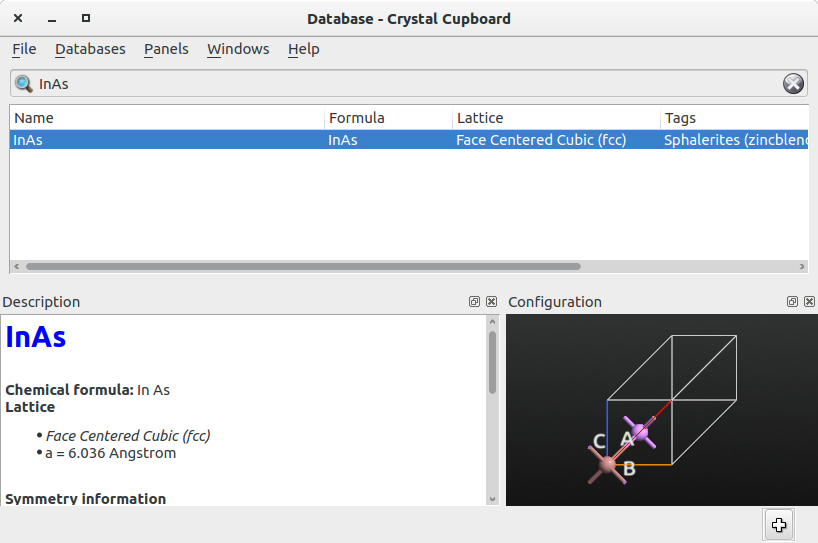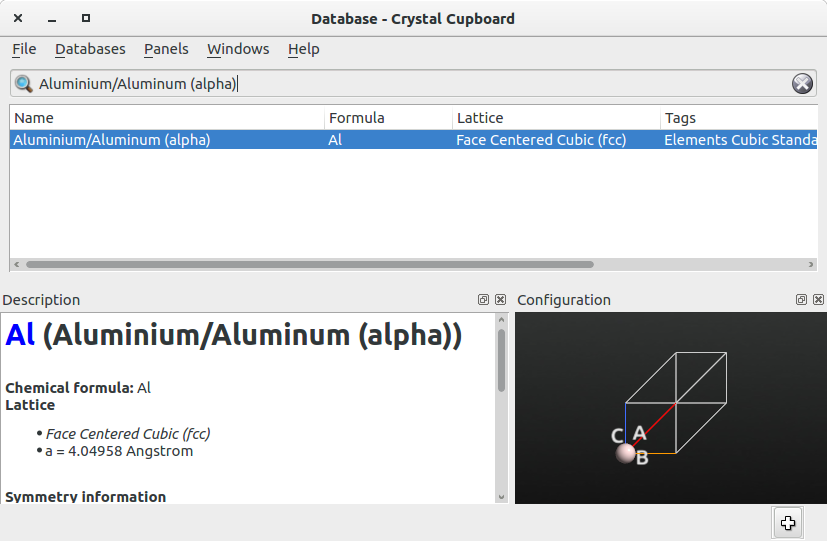# Determination of low strain interfaces via geometric matching¶

Version: 2017.1

This tutorial introduces the new GeneralizedLatticeMatch method for combining two bulk crystals into an interface.

On many occasions, one is interested in creating a realistic interface between two materials, even without having precise structural informations on the two surfaces that form the interface. The GeneralizedLatticeMatch method facilitates this process, by automatically finding all the possible interface supercells between the two crystals based only on their bulk crystalline structure. The method is an optimized version of the algortihm described in [JLS+17]. Compared to the lattice-matching method used in the Interface Builder, described in interface_builder, the present method is unbiased as it considers all the possible surfaces of the two crystals forming the interface.

A number of structural parameters are calculated for each interface, which allows one to easily analyze the matching pattern and choose the most appropriate interface to be used for further studies. The structure of the desired interface can then be easily created using the Interface Builder implemented in QuantumATK.## Method description¶

The following describes in the main steps of the algortihm used in the GeneralizedLatticeMethod method, which is very similar to that used in the Interface Builder (see interface_builder).

1. Initially, the possible surface vectors $$[\mathbf{a}_{1}, \mathbf{a}_{2}]$$ of the first of the two materials forming the interface are constructed, starting from a linear combination of the Bravais vectors of the primitive cell:

\begin{align}\begin{aligned}\mathbf{a}_1 = \sum_{i=1}^3 c_i \mathbf{u}_i \quad c_i \in \mathbb{Z},\\\mathbf{a}_2 = \sum_{i=1}^3 c_i^\prime \mathbf{u}_i \quad c_i^\prime \in \mathbb{Z},\end{aligned}\end{align}

and by a subsequent projection of the resulting vectors from $$\mathbf{R}^3$$ to $$\mathbf{R}^2$$. A similar procedure is applied to construct the surface vectors $$[\mathbf{b}_{1}, \mathbf{b}_{2}]$$ of the second surface. As it will be shown in the Section Input and output description, the number of generated surface cells can be limited by specifying:

• The maximum value of the Miller indexes $$[h,k,l]$$ that define each surface;
• The maximum length of the lattice vectors $$[\mathbf{a}_{1}, \mathbf{a}_{2}]$$ and $$[\mathbf{b}_{1}, \mathbf{b}_{2}]$$.
2. Each couple of surface cells is matched by using the same lattice-match method as used in the Interface Builder, and described in the technical note interface_builder.

3. The average strain is then calculated as:

$\mathbf{\bar{\varepsilon}} = \sqrt{\frac{\varepsilon_{11}^2 + \varepsilon_{22}^2 + \varepsilon_{11}\varepsilon_{22} + \varepsilon_{12}^2}{4}}$

where $$\epsilon_{11}$$, $$\epsilon_{22}$$ and $$\epsilon_{12}$$ are the components of the 2D strain tensor, as defined in the technical note interface_builder.

Note

Notice that this definition of the average strain differs from that used in the interface_builder. The present definition of strain is more appropriate for the present method as it is an invariant of the strain tensor.

## Input and output description¶

In order to use the GeneralizedLatticeMatch method one can set up a simple script as follows:

  1 2 3 4 5 6 7 8 9 10 11 12 13 14 15 16 17 # Read the BulkConfiguration of the primitive cell of the # first material configuration_1 = nlread('InAs.py',BulkConfiguration)[-1] # Read the BulkConfiguration of the primitive cell of the # second material configuration_2 = nlread('Al.py',BulkConfiguration)[-1] # Run the GeneralizedLatticeMatch method generalized_lattice_match = GeneralizedLatticeMatch( configuration_1, configuration_2, max_strain=0.02, maximum_miller_index=3, longest_surface_lattice_vector=50*Angstrom, max_surface_area=200.0*Angstrom**2, user_given_miller_index=None ) 

The script reads two input files, each one containing a BulkConfiguration of the bulk primitive cell of one of the two materials that form the interface. Then, it applies the GeneralizedLatticeMatch method on these two structures. A number of input parameters can be set to control the precision and the extent of the search for possible interface supercells. The full list of input parameters is:

• configuration_1: BulkConfiguration of the bulk primitive cell of the first material.

• configuration_2: BulkConfiguration of the bulk primitive cell of the second material.

• max_strain: Maximum strain applied to each of the two surfaces.

• maximum_miller_index: Maximum value of the Miller indexes $$\left[ h, k, l \right]$$ that define each of the two surfaces.

• longest_surface_lattice_vector: Maximum length of each surface lattice vector $$[\mathbf{a}_{1}, \mathbf{a}_{2}]$$ and $$[\mathbf{b}_{1}, \mathbf{b}_{2}]$$.

• max_surface_area: Maximum value of the surface area of the interface supercell.

• user_given_miller_index: Predefined Miller indexes of the surface of configuration_1.

Note

When the parameter user_given_miller_index is set to a value different than None, the search for the possible surfaces is restricted to the second material, whereas the surface of the first material is kept fixed. This option will be used in Example 2: Lattice match between a bulk system with a predefined surface.

After the possible matches are calculated, the matching results are printed directly in the QuantumATK log file of the calculation. The matches will be listed in order of increasing strain, together with a number of output parameters that characterize each match.

+----------------------------------------------------------------------------------------+
|    A              B        Strain     Atoms    Area    Aspect    Angle    Rotation     |
+----------------------------------------------------------------------------------------+
[ 1  0  0] >-< [ 1  0  0]   0.000110      29     72.9      1.0      90.0        0.0
[ 2  2  1] >-< [ 1  0  0]   0.000110      23    163.9      2.2     153.4       26.6
[ 3  3  2] >-< [ 1  1  1]   0.004030      28    170.9      1.2     162.6       60.3


The output parameters are:

• A: Miller indexes $$\left[h, k, l \right]$$ of the first surface.
• B: Miller indexes $$\left[h, k, l \right]$$ of the second surface.
• Strain: Maximum strain of the two surfaces.
• Atoms: Total number of atoms in the interface supercell.
• Area: Surface area of the interface supercell.
• Aspect: Aspect ratio between two surface vectors of the interface supercell. The ratio is calculated by taking the largest vector at the numerator.
• Angle: Angle between the two vectors of the interface supercell.
• Rotation: Rotation between the two surfaces in the interface supercell.

## Example 1: Lattice match between two bulk systems¶

In this first example, you will calculate the possible matches between indium arsenide and aluminum.

To obtain the structure file containing the BulkConfiguration of bulk InAs, open QuantumATK, go to theBuilder and click on Add ‣ From Database…. In the Database, select the primitive cell of bulk InAs shown in the figure below, add it to the Stash by clicking on thebutton.Repeat the same procedure to add to the Stash the structure of the primitive cell of bulk Al, which is shown in the figure below.In the Stash, save the two configurations by right-clicking on each structure and selecting ‘Save as’. Alternatively, you can download the two files from here: InAs.py, Al.py.

Set up the script for the GeneralizedLatticeMatch method as shown below:

  1 2 3 4 5 6 7 8 9 10 11 12 13 14 15 16 17 # Read the BulkConfiguration of the primitive cell of the # first material configuration_1 = nlread('InAs.py',BulkConfiguration)[-1] # Read the BulkConfiguration of the primitive cell of the # second material configuration_2 = nlread('Al.py',BulkConfiguration)[-1] # Run the GeneralizedLatticeMatch method generalized_lattice_match = GeneralizedLatticeMatch( configuration_1, configuration_2, max_strain=0.02, maximum_miller_index=3, longest_surface_lattice_vector=50*Angstrom, max_surface_area=200.0*Angstrom**2, user_given_miller_index=None ) 

Alternatively, you can download the script from here: match_InAs_Al.py

In this example, the scan will be performed by considering surfaces with a maximum strain of 0.02, with Miller indexes $$h,k,l \leq 3$$, with a maximum length of the lattice vectors of 50 Å, and with a upper threshold for the surface area of 200 Å2.

Run the script in the terminal as atkpython match_InAs_Al.py > match_InAs_Al.log. The output will look as shown below. There are several possible matches, so for briefness only the first 20 matches are shown.

+------------------------------------------------------------------------------+
|                                                                              |
| Atomistix ToolKit 2017.1 [Build ce08f12]                                     |
|                                                                              |
+------------------------------------------------------------------------------+

|--------------------------------------------------|
Miller planes for A    : ==================================================

|--------------------------------------------------|
Miller planes for B    : ==================================================

|--------------------------------------------------|
Matching Miller planes : ==================================================
+----------------------------------------------------------------------------------------+
|    A              B        Strain     Atoms    Area    Aspect    Angle    Rotation     |
+----------------------------------------------------------------------------------------+
[ 1  0  0] >-< [ 1  0  0]   0.000110      29     72.9      1.0      90.0        0.0
[ 2  2  1] >-< [ 1  0  0]   0.000110      23    163.9      2.2     153.4       26.6
[ 3  3  2] >-< [ 1  1  1]   0.004030      28    170.9      1.2     162.6       60.3
[ 3  1  1] >-< [ 2  1  1]   0.004030      10    120.8      1.7      90.0        0.0
[ 3  1  1] >-< [ 3  1  1]   0.004250      16    120.8      1.7     106.8       33.6
[ 3  3  1] >-< [ 3  3  1]   0.004250      16    158.8      2.2     102.9        0.0
[ 3  3  1] >-< [ 3  3  1]   0.005470      13    158.8      2.2     102.9        0.0
[ 3  1  1] >-< [ 3  1  1]   0.005470      13    120.8      1.7     106.8       33.6
[ 2  1  1] >-< [ 2  1  1]   0.005470      17    178.5      2.4      90.0        0.0
[ 2  1  0] >-< [ 2  1  0]   0.005470      13    162.9      1.2     114.1        0.0
[ 1  1  1] >-< [ 1  1  1]   0.005470      13     63.1      1.0     120.0       60.0
[ 1  1  0] >-< [ 1  1  0]   0.005470      17    103.0      1.4      90.0        0.0
[ 1  0  0] >-< [ 1  0  0]   0.005470      13     72.9      1.0      90.0        0.0
[ 1  0  0] >-< [ 2  2  1]   0.005470       7     72.9      1.0      90.0        0.0
[ 3  2  2] >-< [ 1  1  1]   0.005940      23    150.2      1.2     157.6       60.5
[ 1  0  0] >-< [ 2  1  1]   0.006130      19    182.2      3.9      39.8        0.6
[ 3  1  1] >-< [ 1  1  0]   0.007160      18    151.0      1.7      73.2       22.6
[ 3  1  1] >-< [ 1  0  0]   0.007730      19    120.8      1.7      90.0        0.0
[ 3  2  2] >-< [ 3  1  1]   0.008250      13    150.2      2.3     115.9       35.2
[ 1  0  0] >-< [ 1  1  0]   0.008420      28    200.4      2.2      79.7       38.2


Notice how the match with the least strain is that between the $$\langle 100 \rangle$$ surface of InAs and the $$\langle 100 \rangle$$ surface of Al.

## Example 2: Lattice match between a bulk system with a predefined surface¶

In some cases, one could be interested in finding the possible matches of a bulk material to a given surface. In this second example, you will investigate how to find the possible matches of bulk aluminium to the InAs(111) surface. InAs nanowires with $$\langle 111 \rangle$$-oriented facets have been synthesized in Ref. [KZC+15], and it has been demonstrated experimentally that $$\langle 111 \rangle$$-oriented Al layers can be grown on this surface.

Use the same structure files as in Example 1: Lattice match between two bulk systems, and setup the script for the GeneralizedLatticeMatch calculation as follows:

  1 2 3 4 5 6 7 8 9 10 11 12 13 14 15 16 17 # Read the BulkConfiguration of the primitive cell of the # first material configuration_1 = nlread('InAs.py',BulkConfiguration)[-1] # Read the BulkConfiguration of the primitive cell of the # second material configuration_2 = nlread('Al.py',BulkConfiguration)[-1] # Run the GeneralizedLatticeMatch method generalized_lattice_match = GeneralizedLatticeMatch( configuration_1, configuration_2, max_strain=0.02, maximum_miller_index=3, longest_surface_lattice_vector=50*Angstrom, max_surface_area=200.0*Angstrom**2, user_given_miller_index=(1,1,1) ) 

Notice how the user_given_miller_index option, highlighted in yellow, has now been set to user_given_miller_index=(1,1,1). Therefore, in this case only the matches of bulk Al to the InAs(111) surface will be considered. You can also download the script from here: match_InAs111_Al.py

Run the script in the terminal as atkpython match_InAs111_Al.py > match_InAs111_Al.log. The output will look as shown below. Notice how there are fewer possible matches than those in the output of Example 1: Lattice match between two bulk systems. This is because only one surface is now considered for the material denoted A.

+------------------------------------------------------------------------------+
|                                                                              |
| Atomistix ToolKit 2017.1 [Build ce08f12]                                     |
|                                                                              |
+------------------------------------------------------------------------------+

|--------------------------------------------------|
Miller planes for B    : ==================================================

|--------------------------------------------------|
Matching Miller planes : ==================================================
+----------------------------------------------------------------------------------------+
|    A              B        Strain     Atoms    Area    Aspect    Angle    Rotation     |
+----------------------------------------------------------------------------------------+
[ 1  1  1] >-< [ 1  1  1]   0.005470      13     63.1      1.0     120.0       60.0
[ 1  1  1] >-< [ 3  3  1]   0.010100      17    142.0      1.2      46.1       18.4
[ 1  1  1] >-< [ 2  1  0]   0.010710      15    110.4      4.0      30.0        5.9
[ 1  1  1] >-< [ 3  1  1]   0.011070      26    110.4      2.6      19.1       77.9
[ 1  1  1] >-< [ 3  1  1]   0.012770      15    110.4      2.6      19.1       77.9
[ 1  1  1] >-< [ 1  1  0]   0.013260      24    126.2      2.6      40.9       12.1
[ 1  1  1] >-< [ 1  0  0]   0.014880      23    126.2      1.7       8.2        1.1
[ 1  1  1] >-< [ 2  2  1]   0.014880      13    126.2      2.3      10.9       31.1
[ 1  1  1] >-< [ 3  2  1]   0.016000      18    189.3      2.6      26.3       75.4
[ 1  1  1] >-< [ 3  1  2]   0.016000      18    189.3      3.5      19.1       44.6
[ 1  1  1] >-< [ 2  1  0]   0.016150      13    110.4      4.0      30.0        5.9
[ 1  1  1] >-< [ 2  1  1]   0.016420       9     78.9      2.9      30.0       20.8
[ 1  1  1] >-< [ 1  1  0]   0.016510      19    126.2      2.6      40.9       12.1
+----------------------------------------------------------------------------------------+


In this case, the match to the InAs(111) surface with the lowest strain is that with the Al(111) surface, which is in agreement with the experimentally measured structure reported in Ref. [KZC+15].